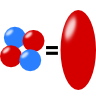## The Quadrupole Moment of a Homogeneously Charged EllipsoidThe quadrupole moments of a homogeneously charged ellipsoid are calculated. The result will help us to understand how and why the quadrupole moments of an ellipsoidal charge distribution changes if it gets into an excited state.

## Problem Statement

1.Determine the quadrupole tensor $Q_{ij}= \int\left(3x_{i}^{\prime}x_{j}^{\prime}-r^{\prime2}\delta_{ij}\right)\rho\left(\mathbf{r}^{\prime}\right)d^{3}\mathbf{r}^{\prime}$ for a homogeneously charged ellipsoid. The domain of the ellipsoid shall be mathematically given by $\Omega = \left\{ x,y,z:\quad\frac{x^{2}}{a^{2}}+\frac{y^{2}}{b^{2}}+\frac{z^{2}}{c^{2}}\leq1\right\}\ ,$ i.e. its principal axes coincide with the coordinate axes.
2. Assume the normalized charge distributions $\begin{eqnarray*} \rho_{\mathrm{ground}}\left(s\right)&=&\tilde{\rho}_{0}\frac{4}{s_{0}^{3}}e^{-2s/s_{0}}\ \mathrm{and}\\\rho_{\mathrm{exc}}\left(s\right)&=&\tilde{\rho}_{0}\frac{1}{3}\frac{1}{\left(2s_{0}\right)^{3}}\left(\frac{s}{s_{0}}\right)^{2}e^{-s/s_{0}} \end{eqnarray*}$for "ground" and "excited" state, respectively. Here, $$s$$ refers to the dimensionless radius variable in ellipsoidal coordinates (see hints). Calculate the ratio of the nonvanishing quadrupole moments with respect to both states.